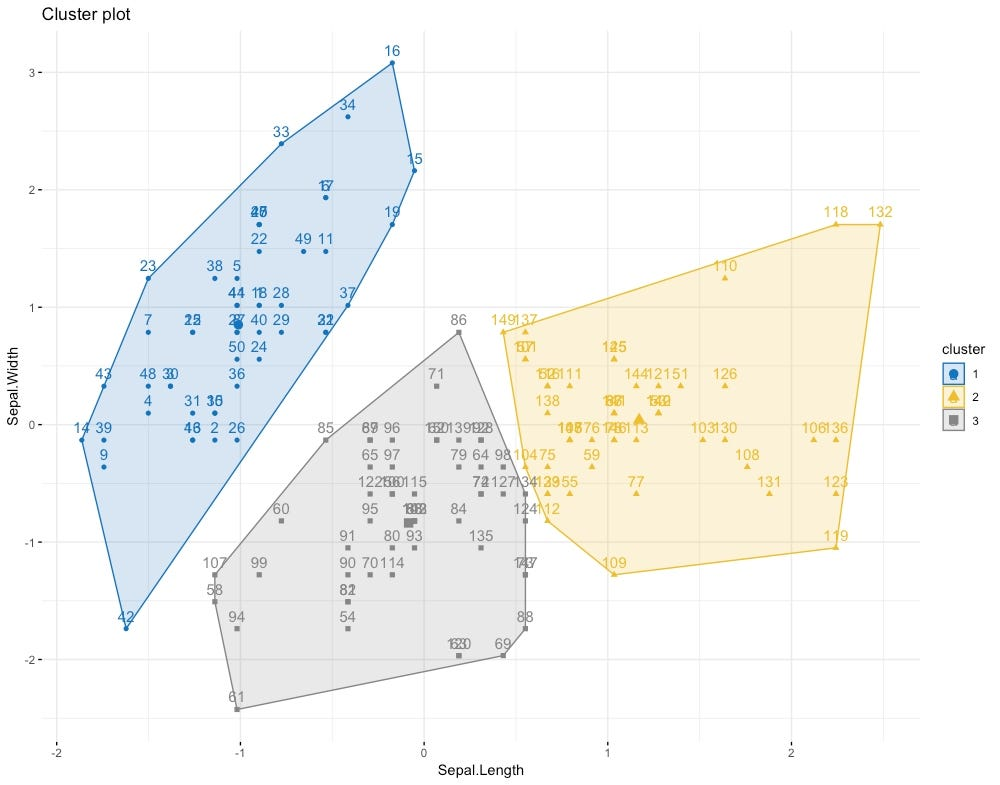Our start with Unsupervised learning was through KNN(K Nearest Neighbor) which is most popular of them all. You can read it here.

Here’s a refinement of it, below is algorithm for PAM(Partitioning Around Mediods)Cool picture of Cluster

Build phase:

1. Select k objects to become the medoids, or in case these objects were provided use them as the medoids;
2. Calculate the dissimilarity matrix
3. Assign every observation to its closest medoid;

Swap phase:
4. For each cluster search if any of the object of the cluster decreases the average dissimilarity coefficient; if it does, select the entity that decreases this coefficient the most as the medoid for this cluster;

5. If at least one medoid has changed go to (3), else end the algorithm.

Points to Note

1. The dissimilarity matrix (also called distance matrix) describes pairwise distinction between M objects. It is a square symmetrical MxM matrix with the (ij)th element equal to the value of a chosen measure of distinction between the (i)th and the (j)th object. The diagonal elements are either not considered or are usually equal to zero.

We can use distance matrix as a dissimilarity matrix, where Euclidean, Manhattan(used when outliers present). Check up this article different options of dissimilarity matrix.

2. K can be selected as described in this article by Elbow Method, Silhouette or Gap Statistics method.

3. Unlike K means where centroid may not be actual observation, clusters of PAM are represented by single member of that cluster where this mediod represent most centrally located points within the cluster.

Now we went through K means, PAM for clustering data, CLARA is another similar method used for large data set. To read more about it visit this.

Resources:

Problem Solver, Data Science, Actuarial Science, Knowledge Sharer, Hardcore Googler

## More from Shaily jain

Problem Solver, Data Science, Actuarial Science, Knowledge Sharer, Hardcore Googler